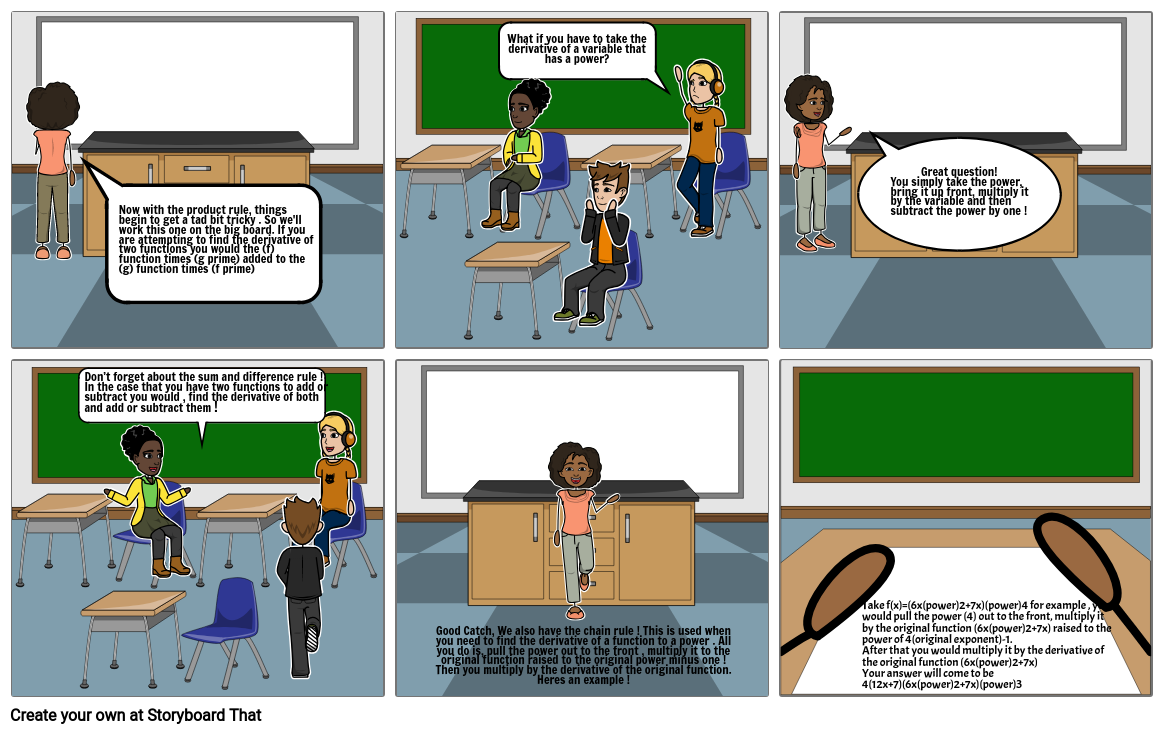calculus project 2More Options: Make a Folding Card

Storyboard Description

This storyboard does not have a description.

Storyboard Text

• Now with the product rule, things begin to get a tad bit tricky . So we'll work this one on the big board. If you are attempting to find the derivative of two functions you would the (f) function times (g prime) added to the (g) function times (f prime)
• What if you have to take the derivative of a variable that has a power?
• Great question! You simply take the power, bring it up front, multiply it by the variable and then subtract the power by one !
• Don’t forget about the sum and difference rule ! In the case that you have two functions to add or subtract you would , find the derivative of both and add or subtract them !
• Good Catch, We also have the chain rule ! This is used when you need to find the derivative of a function to a power . All you do is, pull the power out to the front , multiply it to the original function raised to the original power minus one ! Then you multiply by the derivative of the original function. Heres an example !
• Take f(x)=(6x(power)2+7x)(power)4 for example , you would pull the power (4) out to the front, multiply it by the original function (6x(power)2+7x) raised to the power of 4(original exponent)-1. After that you would multiply it by the derivative of the original function (6x(power)2+7x) Your answer will come to be 4(12x+7)(6x(power)2+7x)(power)3
More Storyboards By d3stinywilliams
Explore Our Articles and Examples

Teacher Resources

Lesson Plans Worksheet TemplatesPoster Templates

Film Resources

Film and Video Resources
Video Marketing